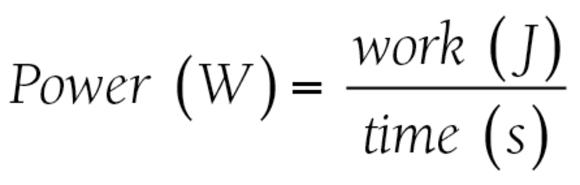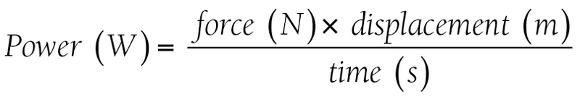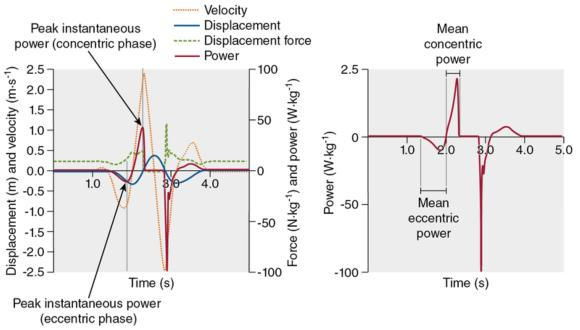Feedback

# Defining the term power in strength and conditioning

### This is an excerpt from Developing Power.

The colloquial use of the term power as a generic trait is commonly misunderstood and interpreted. Power is, by definition, the rate of doing work. The unit of measure for work is the joule and the unit of measure for power is the watt (W), defined as a joule per second. Coaches often indicate that athletes are powerful by describing their movements as occurring at a high velocity relative to the force they must produce or load they must overcome during the movement.Therefore, movements that occur at slower velocities because of external loads that must be moved (e.g., another person during a tackle or a weighted jump squat) may still be described as powerful because the velocity is high relative to the force required or mass being accelerated. The colloquial use of the term powerful was likely a loose interpretation of the mathematical definitions of power. The following mathematical equations associated with power and work can be arranged several ways to derive the various equations for power.Because work is a product of force and displacement, substitution leads to the following equation:Simplified further (because velocity = displacement ÷ time), we can once again rearrange the equation to what is commonly used or expressed by strength and conditioning practitioners as the equation for power:

Power (W) = force (N) × velocity (m/s)

Further, power can be expressed as the mean over the time of the movement, termed mean system power (Pmean), or as the highest peak instantaneous system power (Ppeak). As such, the Pmean will always be a lower value and represents the power across the entire movement, whereas the Ppeak is the power during the highest discrete time point (figure 2.1). For example the Pmean during a countermovement has been reported as 765 W, while Ppeak was reported as 5,014 W.Figure 2.1 A representative countermovement jump with a graphical representation that coincides with standing, lowering during the countermovement, and then extension before takeoff. (a) Displacement, velocity, force, and power versus time with the peak power during both the eccentric and concentric phases of the jump are labeled. Notice that the time at which peak power occurs is not the same time that peak velocity and peak force occur. (b) Power versus time with an indication of what comprises the entire eccentric and concentric phases. The start and finish of each phase is described in full in text.

The current trend in strength and conditioning training to measure and report Pmean or Ppeak has led to the development of ballistic assessments (e.g., bench press throw and jump). During ballistic assessments, power is often calculated to understand the force - velocity profile of an athlete. However, one should understand that ballistic assessments should not be considered the measurement of power. Instead, one may measure Pmean or Ppeak during these ballistic activities. In fact, system power could technically be measured during any activity except for those that are isometric, where velocity is zero and therefore power is zero. Further, when measuring power, it is critical to fully describe the methods of measurement (discussed in the next section) so the results can be interpreted within the correct context. Other variables, such as force and velocity, should also be presented because power is the mechanical construct of force and velocity. Therefore, to correctly interpret power as a measured variable, one must understand the combination of force and velocity changes that elicit the measured power output (see chapter 1 and subsequent section on advantages and drawbacks of power assessment).

Save

Save

Save

Save